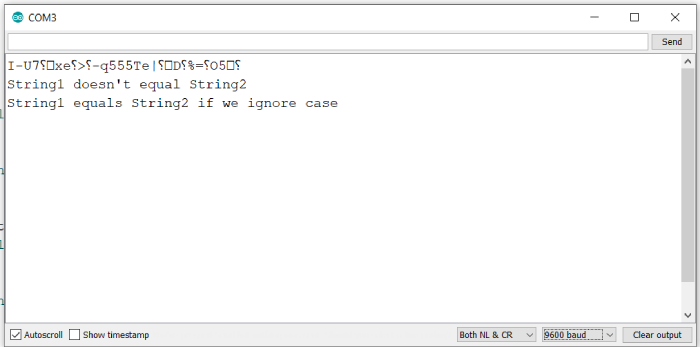# Check if two strings are equal while ignoring case in Arduino

We know that String1.equals(String2) can be used to find out if String1 and String2 are equal in Arduino. However, this function is case sensitive. So, if there is a difference in the case of even a single character, this function will return false. A strategy that people use to perform case insensitive comparison of two strings is to convert both the strings to lower case and then compare. However, Arduino has a function to compare two strings while ignoring case. As you’d have guessed, the function is equalsIgnoreCase.

## Example

An example implementation is given below −

void setup() {
// put your setup code here, to run once:
Serial.begin(9600);
Serial.println();
String String1 = "Hello";
String String2 = "hello";
if (String1.equals(String2)) {
Serial.println("String1 equals String2");
} else {
Serial.println("String1 doesn't equal String2");
}

if (String1.equalsIgnoreCase(String2)) {
Serial.println("String1 equals String2 if we ignore case");
} else {
Serial.println("String1 doesn't equal String2 even if we ignore case");
}
}
void loop() {
// put your main code here, to run repeatedly:
}

## Output

The Serial Monitor output is shown below −As you would have expected, the .equals() function returns false, while the .equalsIgnoreCase() function returns true.